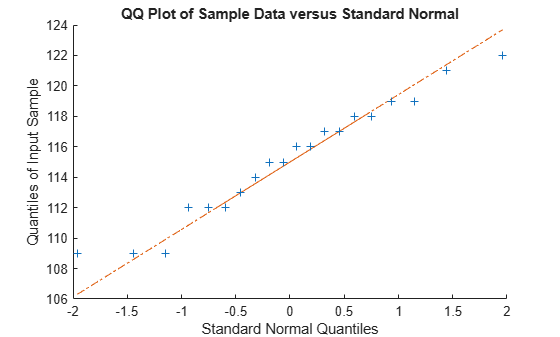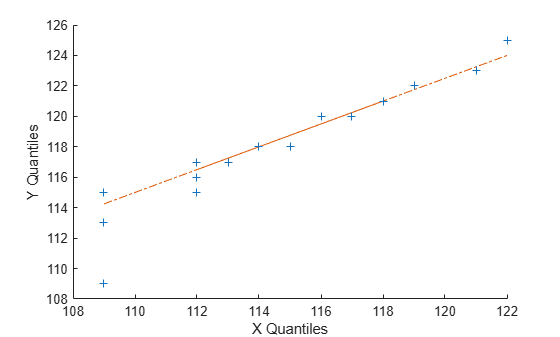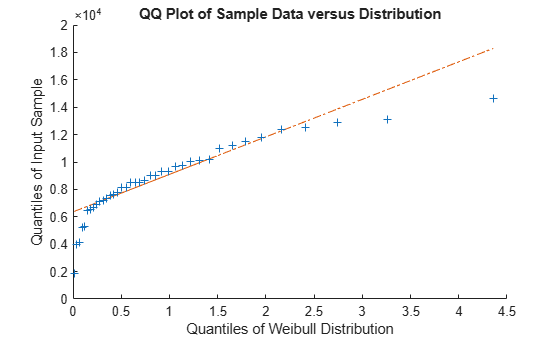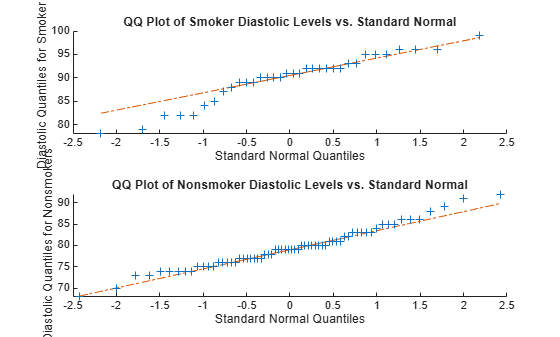# qqplot

Quantile-quantile plot

## Syntax

``qqplot(x)``
``qqplot(x,pd)``
``qqplot(x,y)``
``qqplot(___,pvec)``
``qqplot(ax,___)``
``h = qqplot(___)``

## Description

example

````qqplot(x)` displays a quantile-quantile plot of the quantiles of the sample data `x` versus the theoretical quantile values from a normal distribution. If the distribution of `x` is normal, then the data plot appears linear.`qqplot` plots each data point in `x` using plus sign (`'+'`) markers and draws two reference lines that represent the theoretical distribution. A solid reference line connects the first and third quartiles of the data, and a dashed reference line extends the solid line to the ends of the data.```

example

````qqplot(x,pd)` displays a quantile-quantile plot of the quantiles of the sample data `x` versus the theoretical quantiles of the distribution specified by the probability distribution object `pd`. If the distribution of `x` is the same as the distribution specified by `pd`, then the plot appears linear.```

example

````qqplot(x,y)` displays a quantile-quantile plot of the quantiles of the sample data `x` versus the quantiles of the sample data `y`. If the samples come from the same distribution, then the plot appears linear.```
````qqplot(___,pvec)` displays a quantile-quantile plot with the quantiles specified in the vector `pvec`. Specify `pvec` after any of the input argument combinations in the previous syntaxes.```

example

````qqplot(ax,___)` uses the plot axes specified by the `Axes` object `ax`. The option `ax` can precede any of the input argument combinations in the previous syntaxes.```
````h = qqplot(___)` returns the handles (`h`) to the lines in the quantile-quantile plot.```

## Examples

collapse all

Use a quantile-quantile plot to determine whether gas prices in Massachusetts follow a normal distribution.

`load gas`

The sample data in `price1` and `price2` represent gasoline prices at 20 different gas stations in Massachusetts. The samples were collected during two different months.

Create a quantile-quantile plot to determine if the gas prices in `price1` follow a normal distribution.

```figure qqplot(price1)```The plot produces an approximately straight line, suggesting that the gas prices follow a normal distribution.

Use a quantile-quantile plot to determine whether two sets of sample data come from the same distribution.

`load gas`

The sample data in `price1` and `price2` represent gasoline prices at 20 different gas stations in Massachusetts. The samples were collected during two different months.

Create a quantile-quantile plot using both sets of sample data, to assess whether prices at different times have the same distribution.

`qqplot(price1,price2);`The plot produces an approximately straight line, suggesting that the two sets of sample data have the same distribution.

Use a quantile-quantile plot to determine whether sample data comes from a Weibull distribution.

`load lightbulb`

The first column of the data has the lifetime (in hours) of two types of light bulbs. The second column has information about the type of light bulb. 1 indicates fluorescent bulbs whereas 0 indicates the incandescent bulbs. The third column has censoring information. 1 indicates censored data, and 0 indicates the exact failure time. This is simulated data.

Remove the censored data.

```lightbulb = [lightbulb(lightbulb(:,3)==0,1),... lightbulb(lightbulb(:,3)==0,2)];```

Create a variable for each light bulb type. Include only uncensored data.

```fluo = [lightbulb(lightbulb(:,2)==0,1)]; insc = [lightbulb(lightbulb(:,2)==1,1)];```

Create a Weibull probability distribution object using the default parameters of `A = 1` and `B = 1`.

`pd = makedist('Weibull');`

Create a q-q plot to determine whether the lifetime of fluorescent bulbs has a Weibull distribution.

```figure qqplot(fluo,pd)```The plot is not a straight line, suggesting that the lifetime data for fluorescent bulbs does not follow a Weibull distribution.

Display a side-by-side pair of quantile-quantile plots using the `tiledlayout` and `nexttile` functions.

Load the `patients` data set. Separate the patient diastolic blood pressure levels into two data sets: one containing the diastolic blood pressure levels of smokers and one containing the diastolic levels of nonsmokers.

```load patients smokerIndices = (Smoker == 1); nonsmokerIndices = (Smoker == 0); smokerDiastolic = Diastolic(smokerIndices); nonsmokerDiastolic = Diastolic(nonsmokerIndices);```

Create a 2-by-1 tiled chart layout using the `tiledlayout` function. Create the first set of axes `ax1` within the chart layout by calling the `nexttile` function. In the axes, display a q-q plot to determine whether the diastolic blood pressure levels of smokers come from a normal distribution. Create the second set of axes `ax2` within the tiled chart layout by calling the `nexttile` function. In the axes, display a q-q plot to determine whether the diastolic blood pressure levels of nonsmokers come from a normal distribution.

```tiledlayout(2,1) % Top axes ax1 = nexttile; qqplot(ax1,smokerDiastolic) ylabel(ax1,'Diastolic Quantiles for Smokers') title(ax1,'QQ Plot of Smoker Diastolic Levels vs. Standard Normal') % Bottom axes ax2 = nexttile; qqplot(ax2,nonsmokerDiastolic) ylabel(ax2,'Diastolic Quantiles for Nonsmokers') title(ax2,'QQ Plot of Nonsmoker Diastolic Levels vs. Standard Normal')```The second plot more closely follows a straight line, suggesting that the sample of nonsmoker blood pressure values has an approximately normal distribution. In contrast, the first plot has points below the line to the left, suggesting a heavier tail (more outliers) than a normal distribution.

## Input Arguments

collapse all

Sample data, specified as a numeric vector or numeric matrix. If `x` is a matrix, then `qqplot` displays a separate line for each column.

`qqplot` displays the sample data using the plot symbol `'+'`. A line joining the first and third quartiles of each distribution is superimposed on the plot. The line represents a robust linear fit of the order statistics for the data in `x`. This line is extrapolated out to the minimum and maximum values in `x` to help evaluate the linearity of the data.

Data Types: `single` | `double`

Second set of sample data, specified as a numeric vector or numeric matrix. `x` and `y` do not need to be the same length. However, if `x` and `y` are matrices, they must contain the same number of columns. If `x` and `y` are matrices, then `qqplot` displays a separate line for each pair of columns.

`qqplot` selects the quantiles to plot based on the size of the smaller data set.

Data Types: `single` | `double`

Hypothesized probability distribution, specified as a probability distribution object. `qqplot` plots the quantiles of the input data `x` versus the theoretical quartiles of the distribution specified by `pd`.

Create a probability distribution object with specified parameter values using `makedist`, or fit a probability distribution object to data using `fitdist`.

Quantiles for plot, specified as a numeric value, or vector of numeric values, in the range [0,100].

For a single set of sample data (`x`), `qqplot` uses the quantiles in `x`. For two sets of sample data (`x` and `y`), `qqplot` uses the quantiles in the smaller of the two data sets.

Data Types: `single` | `double`

Axes for the plot, specified as an `Axes` object. If you do not specify `ax`, then `qqplot` creates the plot using the current axes. For more information on creating an `Axes` object, see `axes`.

## Output Arguments

collapse all

Graphics handles for line objects, returned as a vector of `Line` graphics handles. Graphics handles are unique identifiers that you can use to query and modify the properties of a specific line on the plot. For each column of `x`, `qqplot` returns three handles:

• The line representing the data points. `qqplot` represents each data point in `x` using plus sign (`'+'`) markers.

• The line joining the first and third quartiles of each column of `x`, represented as a solid line.

• The extrapolation of the quartile line, extended to the minimum and maximum values of `x`, represented as a dashed line.

To view and set properties of line objects, use dot notation. For information on using dot notation, see Access Property Values. For information on the `Line` properties that you can set, see Line Properties.

collapse all

### Quantile-Quantile Plot

A quantile-quantile plot (also called a q-q plot) visually assesses whether sample data comes from a specified distribution. Alternatively, a q-q plot assesses whether two sets of sample data come from the same distribution.

A q-q plot orders the sample data values from smallest to largest, then plots these values against the expected value for the specified distribution at each quantile in the sample data. The quantile values of the input sample appear along the y-axis, and the theoretical values of the specified distribution at the same quantiles appear along the x-axis. If the resulting plot is linear, then the sample data likely comes from the specified distribution.

The q-q plot selects quantiles based on the number of values in the sample data. If the sample data contains n values, then the plot uses n quantiles. Plot the ith ordered value (also called the ith order statistic) against the $\frac{i-0.5}{n}$th quantile of the specified distribution.

A q-q plot can also assess whether two sets of sample data have the same distribution, even if you do not know the underlying distribution. The quantile values for the first data set appear on the x-axis and the corresponding quantile values for the second data set appear on the y-axis. Since q-q plots rely on quantiles, the number of data points in the two samples does not need to be equal. If the sample sizes are unequal, the q-q plot chooses the quantiles based on the smaller data set. If the resulting plot is linear, then the two sets of sample data likely come from the same distribution.

## Version History

Introduced before R2006a